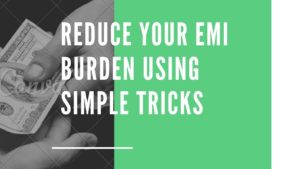Every body who bought a house or planning to buy one on a mortgage, always try to look for ways to reduce his or her monthly obligations i.e. EMI of home loan, there are many ways of doing this, in this article we will focus on some of the simple ones.Reduce home laon EMI

Before getting on to the topic of how to reduce the EMI of home loan, let us first understand what are factors that decide the EMI.
Quantitative factors
Quantitative factors can be summarized by the following equation

Where

C= cost of the asset(house)
D = down payment
n = tenure of loan or loan period( months in case of housing loans)
r = interest rate per period ( monthly in case of housing loans) = APR/p
APR = applicable annual percentage rate of interest
p = payment frequency ( generally monthly in case of home loans)

Don’t worry !, we are not going to into the jargon of equations, rather we will understand this with the help of numbers and CRUX of relationships between various factors affecting the loan EMI based on the above Formula.
CRUX:
1. Down payment (D) is inversely related with the EMI i.e. as the Down payment increases, EMI decreases.
2. Interest rate(APR) and EMI are directly related i.e. if the the interest rate is higher for the loan, EMI will also be higher.
3. Payment frequency(p) and EMI are inversely related, i.e. for higher Payment frequency, EMI is lower.
4. Laon term or tenure is inversely related with the EMI, i.e. for the higher loan term, EMI will be lower.
Let’s understand these with the help of an
Example.
Mr. Lee wants to buy a house that costs 60,00,000, A bank is offering a mortgage at an APR of 8% for 30 years with a minimum down payment of 10% and monthly installments.
Now let us calculate the EMI for this loan,
Using this free calculator

Our down payment is = 10% of 6,000,000 = 600,000. So we need to borrow 5,400,000. As we can see our EMI is 39624 Total interest interest in all 30 * 12 = 360 installments is 8,864,383 too big no ! Even bigger than our loan amount It is because of longer loan term.

Now let us come to our topic as to how to reduce the emi of home loan,

1. Down payment:
If we increases our down payment, We can reduce our emi to some extent let say instead of minimum 600,000, we pay 700,000, then our loan amount will be 5,300,000 and EMI will be is 38,890

however it depends upon the current financial and liquidity position whether he/she can pay higher down payment or not. But it is always advisable to pay higher down to not only reduce the emi but also avoid the higher interest burden as we can see our total interest reduced to 8,700,228

2. Loan term
As we have seen above, that for longer loan term, EMI is lower but it comes with an issue attached i.e. if we get our loan term increased, overall interest burden also increases and vice versa, let see how
Increase loan term to 32 years

EMI becomes 39,044
And overall interest burden rises upto 9,592,911 or 78% more than our loan amount itself, horrifying !
And let reduce the term to 25 years

EMI increases upto = 41,678.08 but overall interest burden decreases to 7,103,422.75
so here is the irony, we need to decrease the EMI, without increasing overall interest burden, this can be done using excel’s solver.

3. Interest rate
Since interest rates are directly related to the EMI, we need a lower interest rate to get the EMI reduced.
3.1For existing mortgage obligations:
3.1.1.Floating rate laon:
In case of floating rate liability, except public banks, every other bank adjust the lending rate, when interest rates increases in the market but don’t reduce it when interest rates decreases, borrower need to apply for reduction in the rates, infact some private banks and NBFCs bypass the monetary policy by adjusting their lending spreads, because the lending rate = WALR + spread, when repo rate is reduced by RBI in its monetary policy, WALR is required to be reduced by the banks and banks do the same but they increase their spread because of which lending rates remains unchanged, in such case the only option left is prepayment or takeover i.e. prepaying the higher interest loan by borrowing at lower rate from a different bank.

3.1.2 Fixed rate loan :
Where interest rate are rising in the market, nothing need to be done, as the your existing obligation is at the lowest possible interest.
However when the interest rates are falling then interest rates can be reduced using takeover(though it’s a different thing that takeover involves some additional cost).

3.2 New loanIn new loan, borrowers have the options to opt for lowest interest rate available, there are various websites which provides comparative analysis of loans offered by various banks and other financial institutions, but to compare those offers, interest rates needs to be converted into effective annual rate, which can be done using this free calculator. However the final lending rate depends on various other factors, like existing debt level of the borrower, repayment capacity, default risk etc.
Now you can also calculate your own EMI either by using the above mentioned formula or the following alternative formula

Where
C = cost of asset or house
D = down payment
n = loan tenure
r = APR or interest rates
P = frequency
Or you can use this free EMI calculator